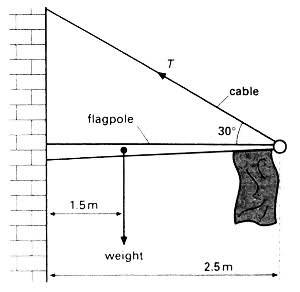# Moments and finding the Tension-Help

## Homework Statement

1. Homework Statement

A flagpole of mass 25kg and 2.5m in length is held in a horizontal position by a cable shown in the diagram.
The centre of gravity of the flagpole is at a distance of 1.5m from the fixed end. Determine:
a the tension T in the cable;
b the vertical component of the force at the fixed end of the pole.## Homework Equations

Moment=F*perp distance
vertical component=Fsin(theta)
Horizontal component=Fcos(theta)
clockwise=anticlockwise

## The Attempt at a Solution

I did (a) and I got around 300.Please call out on me if i get this wrong.
Calculate the moments around the end of the flagpole(left hand)
clockwise moment=250*1.5=375
anti clockwise moment=T*1.25
through division etc, i get 300.

What i cannot understand is (b) What does it mean by the vertical component at the end of the flagpole?

## Answers and Replies

SteamKing
Staff Emeritus
Science Advisor
Homework Helper
What happens when you replace T with its components? BTW, your moment calculation involving T is incorrect.